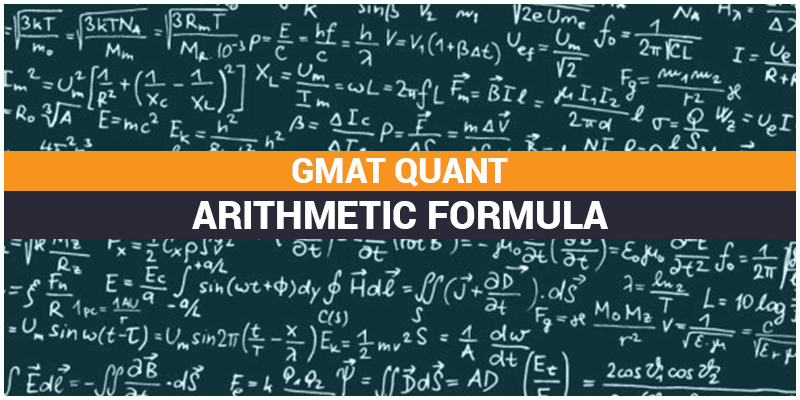# GMAT Quant: Arithmetic FormulaOther than Algebra and Geometry, GMAT Quant consists sums oriented to numbers, traditional operations between them like addition, subtraction, multiplication and division. Some major Arithmetic topics & their formulas are as follows –

## Combinatorics – Factorials, Permutations & Combinations

Factorials –

x factorial = x! = x (x-1) (x-2) … (3) (2) (1) (or)

n! = 1.2.3 …. (n-2) (n-1) n

0! = 1

Permutations –

$_{n}P_{k} = \frac{n!}{(n-k)!}$

Where, n = the number of objects to choose from and k = the number of objects selected

Combinations –

$_{n}C_{k} = \frac{n!}{(n-k)!k!}$

Where, n = the number of objects to choose from and k = the number of objects selected

Permutations & Combinations

Permutations – $^{a}P_{b}$

Combinations – $^{a}C_{b}$

Where, a, b are Whole Numbers

Some Important Combinatorics Formulas

1. $^{n}P_{n} = n!$
2. $^{n}P_{m} = \frac{n!}{(n-m)!}$
3. Binomial Coefficient

$^{n}C_{m} = \frac{n}{m} = \frac{n!}{m! (n-m)!}$

1. $^{n}C_{m} = \; ^{n}C_{n-m}$
2. $^{n}C_{m} + \; ^{n}C_{m+1} = \; ^{n+1}C_{m+1}$
3. $^{n}C_{0} + ^{n}C_{1} + ^{n}C_{2} + ….. + ^{n}C_{n} = 2^{n}$

## Fractions

$\frac{a}{b} + \frac{c}{d} = \frac{(ad + bc)}{bd}$

Subtracting Fractions –

$\frac{a}{b} – \frac{c}{d} = \frac{(ad – bc)}{bd}$

Multiplying Fractions –

$\frac{a}{b} \times \frac{c}{d} = \frac{ac}{bd}$

Dividing Fractions –

$\frac{\frac{a}{b}}{\frac{c}{d}} = \frac{a}{b} \div \frac{c}{d} = \frac{a}{b} \times \frac{d}{c} = \frac{ad}{bc}$

Formula for a proportion –

$\frac{a}{b} = \frac{c}{d}$

In a proportion, the product of the extremes (ad) equal the product of the means(bc), Thus, ad = bc

## Percents

Converting Percentage into Decimal

$20 \% = \frac{20}{100} = 0.5$

Converting Decimal Into Percentage

$0.25 = (0.25 \times 100) \% = 25 \%$

$1.50 = (1.50 \times 100) \% = 150 \%$

Percent Change

$Percent \; Change = \frac{(End \; Value) – (Start \; Value)}{(Start \; Value)} * 100$

$Percent \; Change (as \; decimal)= \frac{(End \; Value) – (Start \; Value)}{(Start \; Value)}$

Percentage Increase/Decrease

If the price of a commodity increases by R%, then the reduction in consumption so as not to increase the expenditure is:

$\left [ \frac{R}{(100 + R)} \times 100 \right ]\%$

If the price of a commodity decreases by R%, then the increase in consumption so as not to decrease the expenditure is:

$\left [ \frac{R}{(100 – R)} \times 100 \right ]\%$

Percentage Formula for Population Sums

For Present Population P and suppose it increases at the rate of R% per annum, then:

$Population \; after \; n \; years = P \left ( 1 + \frac{R}{100} \right )^{n}$

$Population \; n \; years \; ago = \frac{P}{\left ( 1 + \frac{R}{100} \right )^{n}}$

Shortcuts to solve Percentage

It is Advisable to memorize the following values. It will save your while solving GMAT Quant –

 Percent Decimal Fraction $1\%$ 0.01 $\frac{1}{100}$ $5\%$ 0.05 $\frac{1}{20}$ $10\%$ 0.1 $\frac{1}{10}$ $12\frac{1}{2}\%$ 0.125 $\frac{1}{8}$ $20\%$ 0.2 $\frac{1}{5}$ $25\%$ 0.25 $\frac{1}{4}$ $33\frac{1}{3}\%$ 0.333 $\frac{1}{3}$ $50\%$ 0.5 $\frac{1}{2}$ $75\%$ 0.75 $\frac{3}{4}$ $80\%$ 0.8 $\frac{4}{5}$ $90\%$ 0.9 $\frac{9}{100}$ $99\%$ 0.99 $\frac{99}{100}$ $100\%$ 1 $125\%$ 1.25 $\frac{5}{4}$ $150\%$ 1.5 $\frac{3}{2}$ $200\%$ 2

We’ll be glad to help you in your GMAT preparation journey. You can ask for any assistance related to GMAT and MBA from us by calling us at +918884544444. You can write to us at gmat@byjus.com.